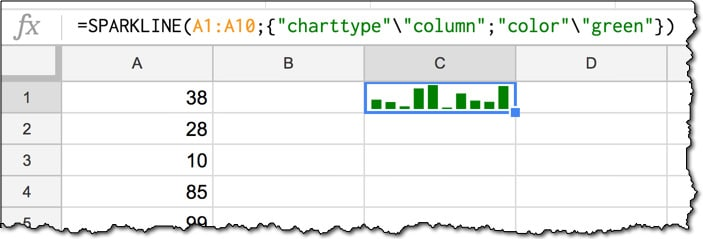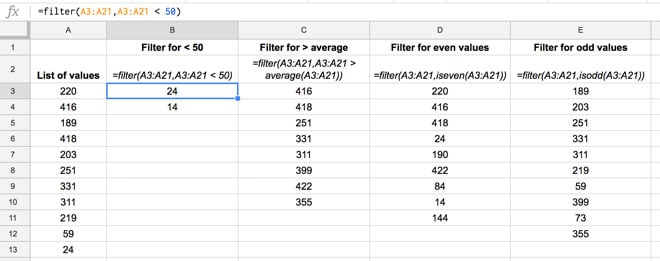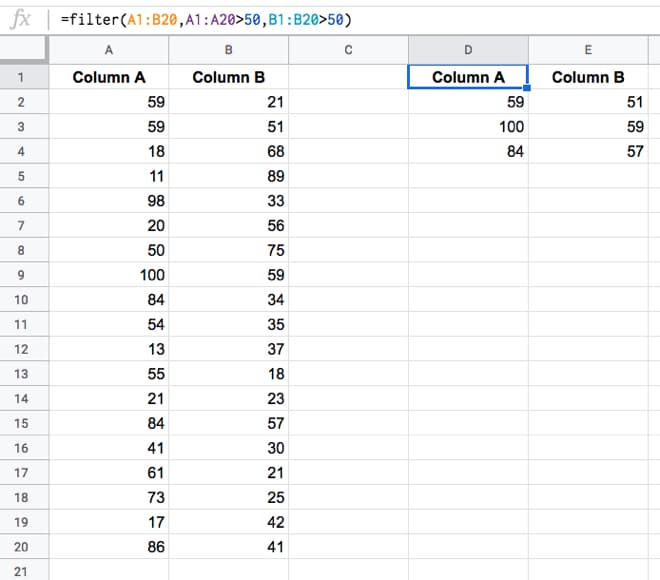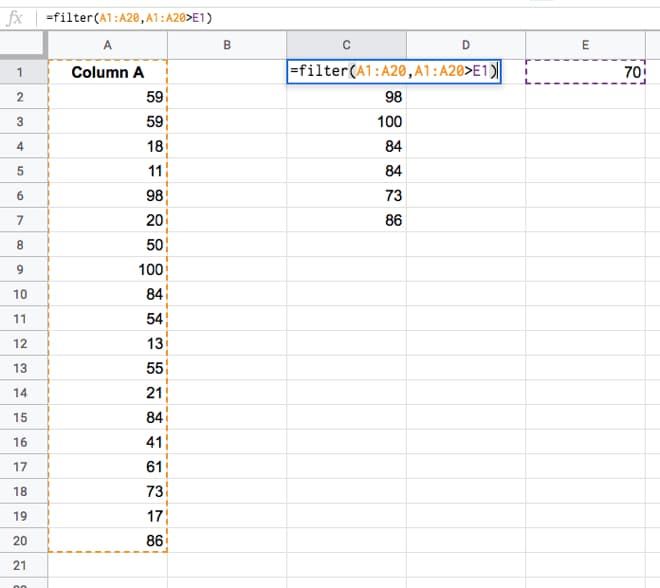Did you know that formulas are written differently depending on where in the world you’re located? For example, the syntax in the US is different to that in Italy.

This post explores the syntax differences that occur based on your Google Sheets location, i.e. the location you’re working in, assuming your Google settings match (which they would by default).What wizardry is this? Either this format will look utterly normal to you, or it won’t.

If you’ve ever copied a template but been unable to get it working, or simply not understood a formula, then it’s possible you’ve run into this syntax issue due to Google Sheets location.

This handy guide will show you the differences and hopefully help you translate seamlessly when sharing Sheets in different locations.

For the most of the world, aside from Europe, you write decimals with a decimal point notation (for example `\$2.50`) and your formulas will use commas to separate the different parts.

I’m currently based in the US, my Google account is set to a US location, so all the articles and template downloads on this site use this notation. (Incidentally, I’m from the UK originally, but since they use the same decimal notation there, formulas in my Google Sheets are the same regardless.)

For countries using decimal comma separators (for example `€2,50`), which is most of the European countries and a select few others, the syntax for formulas is slightly different, as explained below.

So, ask yourself now where you’re based and how you write your decimal numbers, and then see the different sections below for guidance on how your formulas are written.

## How to change Google Sheets location

Before getting to the nitty-gritty of formula syntax, let’s first see where we set the location.

## A Guide To The Google Sheets Filter Function

The Google Sheets Filter function is a powerful function we can use to filter our data. The Google Sheets Filter function will take your dataset and return (i.e. show you) only the rows of data that meet the criteria you specify (e.g. just rows corresponding to Customer A).

Suppose we want to retrieve all values above a certain threshold? Or values that were greater than average? Or all even, or odd, values?

The Google Sheets Filter function can easily do all of these, and more, with a single formula.

This video is lesson 13 of 30 from my free Google Sheets course: Advanced Formulas 30 Day Challenge.

## What is the Filter function?

In this example, we have a range of values in column A and we want to extract specific values from that range, for example the numbers that are greater than average, or only the even numbers.

The filter formula will return only the values that satisfy the conditions we set. It takes two arguments, firstly the full range of values we want to filter and secondly the conditions we’re going to apply. The syntax is:

`=FILTER("range of values", "condition 1", ["condition 2", ...])`

where Condition 2 onwards are all optional i.e. the Filter function only requires 1 condition to test but can accept more.

## How do I use the Filter function in Google Sheets?For example in the image above, here are the conditions and corresponding formulas:

 Conditions Formula Filter for < 50 `=filter(A3:A21,A3:A21<50)` Filter for > average `=filter(A3:A21,A3:A21>AVERAGE(A3:A21))` Filter for even values `=filter(A3:A21,iseven(A3:A21))` Filter for odd values `=filter(A3:A21,isodd(A3:A21))`

The results are as follows:(Note: not all the values are shown in column A.)

Grab the data and solution file for this tutorial:

### Can I test multiple conditions inside a Google Sheets FILTER function?

Absolutely!

For example, using the basic data above, we could display all the 200-values (i.e. values between 200 and 300) with this formula:

`=FILTER(A3:A21, A3:A21>200, A3:A21<300)`

### Can I test multiple columns in a Filter function?

Yes, simply add them as additional criteria to test. For example in the following image there are two columns of exam scores. The Filter function used returns all the rows where the score is over 50 in both columns:The formula is:

`=FILTER(A1:B20,A1:A20 > 50,B1:B20 > 50)`

Note, using the Filter function with multiple columns like this demonstrates how to use AND logic with the Filter function. Show me all the data where criteria 1 AND criteria 2 (AND criteria 3...) are true.

### Can I reference a criteria cell with the Filter function in Google Sheets?

Instead of hard-coding a value in the criteria, you can simply reference another cell which contains the test criteria. That way you can easily change the test criteria or use other parts of your spreadsheet analysis to drive the Filter function.

For example, in this image the Filter function looks to cell E1 for the test criteria, in this case 70, and returns all the values that exceed that score, i.e. everything over 70.The formula in this example is:

`=FILTER(A1:A20,A1:A20 > E1)`

### Can I do a filter of a filter?

Yes, you can!

Use the output of your first filter as the range argument of your second filter, like this:

`=FILTER( FILTER( range, conditions ), conditions )`